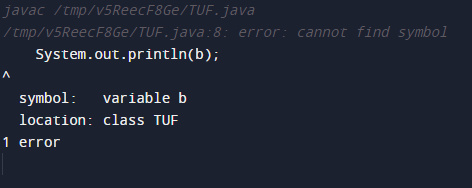# Java expressions, statements, and blocks

### Introduction

Any programming language comprises generally these 3 components which are expressions, statements, and blocks. Without having proper knowledge of expressions one won’t understand how a statement is working and block helps in understanding the scope of the code.

Let’s try understanding these 3 components for a better understanding of JAVA.

### Java Expressions

In Java, expressions are very similar to mathematical expressions but they also include method calls. A java expression is generally made up of variables and operators. For example

```int result = 0; // expression 1
result = res + 1; // expression 2```

Expressions generally return a single value and this value totally depends on the elements which are used within that expression. This returned value can be of any type varying from int to String and Boolean.

For example,

```int a = 10;
Boolean val = true;```

Here the first expression would return an int type value since the assignment operator is assigning a value to a variable that is of int data type. Similarly, the second expression would return a Boolean-type value.

Considering the mathematical expressions in java, we can construct complex mathematical expressions keeping in mind the precedence level of each operand. For example,

```a + b + c + d / e  // expression 1
(a + b + c + d) / e // expression 2```

Here the above two expressions would look similar but they won’t return the same value since the “/” operator has higher precedence as compared to the “+” operator.

Generally, there exists no order while evaluating the expressions. It totally depends on the precedence level of the operators which are used within an expression.

It is always recommended to use brackets whenever you are dealing with complex mathematical expressions or expressions which involve multiple operators.

### Java Statements

Any line of code is a statement. Generally, a line of code can be converted to a statement by terminating it with “;”.

For example,

```int a = 10;
a++;
System.out.println(a);```

Generally, there are 3 types of statements that exist in Java. They are as follows:

1. Expression statements.
2. Declarative statements.
3. Control Flow statements.

Let’s discuss this one by one.

### Expression statements

These types of statements include method calls, mathematical expressions, object creation, etc.

For example,

```a = 234;
a++;
System.out.println(a);```

Here all the lines in the above example are expression statements.

Statement 1: Assignment statement.

Statement 2: Increment statement.

Statement 3: Method calls.

Suppose we have a class named myClass and we want to create an object. We can do it in the following way:

`myClass obj = new myClass();`

The above line is also an expression statement.

### Declarative Statement

Generally, the variable declaration is known as a declarative statement.

For example,

```Boolean val;
int a;```

### Control Flow Statement

Generally, the given code is executed from top to bottom but one can easily change the flow of this execution by using control flow statements. These control flow statements are divided into 3 parts which are as follows:

1. Decision Statements.
2. Looping statements.
3. Branching statements.

Decision statements include if-else and switch-case statements.

Looping statements include for, while, and do while statements.

Branching statements include break, continue and return statements.

### Blocks

Blocks are generally defined using curly braces “{}”. They are used to define the scope of a given set of codes.

For example,

Code:

## Java Code

``````class TUF { // block 1
public static void main(String[] args) { //block 2
int a = 10;
if (a == 10) { // block 3
int b = 3;
System.out.println(a + b);
}
}
}
``````

Output: 13

If we talk about the scope of variables in the blocks, variable a is accessible throughout the main method whereas variable b is defined within the if block hence it cannot be accessed out of the if block. If we try to do it, it would result in an error.

Code:

## Java Code

``````class TUF {
public static void main(String[] args) {
int a = 10;
if (a == 10) {
int b = 3;
System.out.println(a + b);
}
System.out.println(b); // checking for accessibility
}
}
``````

Output:Blocks can be used to define the validity of an expression, method, or variable declared within the method.